# Abstracts

Bed aerators designed to increase air void ratio are used to prevent cavitation and related damages in spillways. Air entrained in spillway discharges also increases the dissolved oxygen concentration of the water, which can be important for the downstream fishery. This study considers results from a systematic series of measurements along the jet formed by a bed aerator, involving concentration profiles, pressure profiles, velocity fields and corresponding air discharges. The experimental results are, then, compared, with results of computational fluid dynamics (CFD) simulations with the aim of predicting the air discharge numerically. Comparisons with jet lengths and the air entrainment coefficients from the literature are also made. It is shown that numerical predictive tools furnish air discharges comparable to measured values. However, if more detailed predictions are desired, verification experiments are still necessary.

spillway aerators; air entrainment; air-water flows; multiphase flows

Aeradores de fundo projetados para aumentar a concentração de ar são utilizados para previnir a cavitação e danos dela derivados em vertedouros. O oxigênio contido na água também é um parâmetro relevante para garantir alta qualidade das águas a jusante do vertedouro, com reflexos na qualidade ambiental. Equações e critérios de projeto existentes ainda são considerados aproximados, mostrando a necessidade de mais estudos para elucidar os mecanismos que governam o carreamento de ar. Este trabalho apresenta resultados de uma série sistemática de medidas de concentração de ar ao longo da superfície inferior do jato de um aerador de fundo, juntamente com medidas pertinentes de descargas de ar e campos de velocidade da água. Foram feitas comparações com resultados da literatura, considerando perfis de concentração ao longo do jato do aerador até a região de jusante. As medições sob condições controladas forneceram informações necessárias para testar resultados numéricos de aeração obtidos em simulações desses escoamentos, utilizando mecânica dos fluidos computacional (CFD). Mostra-se que ferramentas numéricas preditivas fornecem vazões de ar comparáveis aos valores medidos. Também é concluído que, se detalhes são necessários, experimentos são ainda úteis.

aeradores de vertedouros; carreamento de ar; escoamento ar-água; escoamentos multifásicos

ENGINEERING SCIENCES

Lower nappe aeration in smooth channels: experimental data and numerical simulation

Eudes J. ArantesI; Rodrigo M. PortoII; John S. GulliverIII; Alberto C.M. LimaIV; Harry E. SchulzII, V

IUTFPR, Campus Campo Mourão, Caixa Postal 271, 87301-005 Campo Mourão, PR, Brasil

IIDepartamento de Hidráulica e Saneamento/EESC/USP, Av. Trabalhador São-carlense, 400, 13566-590 São Carlos, SP, Brasil

IIIDepartment of Civil Engineering, University of Minnesota, 2 Third Avenue S.E, 55455 Minneapolis, MN, USA

IVUniversidade da Amazônia, Av. Alcindo Cancela, 287, Umarizal, 66060-000 Belém, PA, Brasil

VNúcleo de Engenharia Térmica e Fluidos/EESC/USP, Av. Trabalhador São-carlense, 400, 13566-590 São Carlos, SP, Brasil

Correspondence to

ABSTRACT

Bed aerators designed to increase air void ratio are used to prevent cavitation and related damages in spillways. Air entrained in spillway discharges also increases the dissolved oxygen concentration of the water, which can be important for the downstream fishery. This study considers results from a systematic series of measurements along the jet formed by a bed aerator, involving concentration profiles, pressure profiles, velocity fields and corresponding air discharges. The experimental results are, then, compared, with results of computational fluid dynamics (CFD) simulations with the aim of predicting the air discharge numerically. Comparisons with jet lengths and the air entrainment coefficients from the literature are also made. It is shown that numerical predictive tools furnish air discharges comparable to measured values. However, if more detailed predictions are desired, verification experiments are still necessary.

Key words: spillway aerators, air entrainment, air-water flows, multiphase flows.

RESUMO

Aeradores de fundo projetados para aumentar a concentração de ar são utilizados para previnir a cavitação e danos dela derivados em vertedouros. O oxigênio contido na água também é um parâmetro relevante para garantir alta qualidade das águas a jusante do vertedouro, com reflexos na qualidade ambiental. Equações e critérios de projeto existentes ainda são considerados aproximados, mostrando a necessidade de mais estudos para elucidar os mecanismos que governam o carreamento de ar. Este trabalho apresenta resultados de uma série sistemática de medidas de concentração de ar ao longo da superfície inferior do jato de um aerador de fundo, juntamente com medidas pertinentes de descargas de ar e campos de velocidade da água. Foram feitas comparações com resultados da literatura, considerando perfis de concentração ao longo do jato do aerador até a região de jusante. As medições sob condições controladas forneceram informações necessárias para testar resultados numéricos de aeração obtidos em simulações desses escoamentos, utilizando mecânica dos fluidos computacional (CFD). Mostra-se que ferramentas numéricas preditivas fornecem vazões de ar comparáveis aos valores medidos. Também é concluído que, se detalhes são necessários, experimentos são ainda úteis.

Palavras-chave: aeradores de vertedouros, carreamento de ar, escoamento ar-água, escoamentos multifásicos.

INTRODUCTION

Bottom aerators are a technique used to prevent cavitation erosion on spillways and to enhance the oxygen content of the water. Air vented through the bottom aerators is entrained into the flowing water, increasing the compressibility of the air-water mixture and lowering the velocity of pressure waves. When implosion of cavitation bubbles occurs, the higher compressibility of the surrounding fluid dampens the impact of the pressure waves. Additionally, the bubbles increase the contact area between air and water, improving the oxygen dissolution into the water and the DO content downstream of the spillway.

Experimental investigations on spillway air entrainment by bottom aerators have resulted in empirical design equations. Schwarz and Nutt (1963) presented a theoretical equation for the jet length formed after the ramp. Pan et al. (1980) and Pinto et al. (1982) related the air discharge to geometrical parameters of the jet. Additionally, Tan (1984) and Rutschmann and Hager (1990) explained the dependence of the air discharge on the jet length. The jet length predictions obtained by Tan (1984) are close to those of Schwarz and Nutt (1963).

In Brazil, the studies on aerated spillways were intensified during the construction of the hydropower dams in the 1970 and 1980 decades. The first relevant conclusions for spillways were presented by Pinto et al. (1982), while Borsari (1986) and Fuentes (1992) furnished reviews of important studies and procedures. These aerator studies helped in the establishment of locally adopted procedures. Some early studies, like Volkart (1980) and Wood (1985), added important conceptual contributions for the understanding of aerated flows. Practical applications of the research results, however, require more detailed measurements and the review of existent results. Kökpinar and Gögüs (2002) conducted an extensive experimental study and furnished correlations not only for the jet length, but also for the air entrainment in lower and upper nappes, and a graphical presentation of the effect of ramp heights and bed slopes. The redistribution of flow velocity in the aeration zone was considered by Toombes and Chanson (2005), while the details of the geometry of the air-water interface were used to propose the concept of "entrapped air" by Wilhelms and Gulliver (2005). A similar entrapped air concept was used by Lima et al. (2008) to explain measurements of air void ratios in lower nappe aeration.

This paper seeks to compare CFD simulations with a detailed experimental study of the lower nappe of a jet generated by an aerator in a laboratory chute. Measurements of velocity fields, pressure and air concentration (void ratio) profiles will be compared to CFD results. The CFD simulations of air discharges were comparable to the measured values. It is also shown that, if a more detailed description is needed, experiments are still necessary.

EXPERIMENTAL METHODS

The experiments were conduced in a chute built in the Laboratory of Environmental Hydraulics of the School of Engineering at São Carlos, Brazil. The chute had a slope of 14.5°, an useful length of 5.0 m, with a rectangular cross section 0.20 m wide and 0.50 m high. The bed aerator was composed of a ramp with a length of 23.0 cm, a final height tr = 4.0 cm and an angle of 10° relative to the chute. The chamber under the jet had a depth of 12.0 cm, a length of 18.0 cm and a width of 20.0cm. The air discharge was measured in the air supply tube, which had a diameter of 71.65mm. Air velocities were obtained from pressure measurements, with a micromanometer having one side opened to the atmosphere and the other fixed in a pre-calibrated position in the tube. The concentration measurements in the flow were made with a Cesium 137 probe, as shown in Figure 1. Calibration was made with the channel a) full with water and b) empty. The Cesium 137 radiation was projected perpendicularly to the flow in the channel and a counter registered the remaining radiation after passing the mixture of air and water and the glass walls. The concentration measurements thus consider the entire width of the channel. Air concentration profiles were obtained by positioning the probe and radiation counter along the vertical of every studied cross section. Each obtained concentration is a horizontal mean value transverse to the flow direction.

An electromagnetic flow meter was used to measure the water discharges, which were checked with a rectangular weir located at the outlet of the channel. The measurement of velocity fields in the jet was performed for nine runs, using a mirror inside of the flow. Velocity fields were measured using particle image velocimetry (PIV). The light source was a copper gas Laser, with a mean power of 20 W and pulses at 10 kHz. The pulse power ranged from 60 to 140 kW. Generated wavelengths were 510.6 nm (green) and 578.2 nm (yellow). A CCD camera with a resolution of 1024 pixels x 1024 pixels was used to record the images. After capturing and storing the images in the computer, PIV software was applied to each image to obtain velocity vector fields by using auto-correlation calculations. 234 images were taken for each run. Figure 2 shows all cross sections used in the present study. Sections 1, 2 and 3 were used to obtain the approach flow information (velocity and water depth). Sections 4 through 8 were used for the concentration measurements. Additional sections SIX and SX were used for velocity measurements in the jet core. For velocity measurements in sections 1 and 2, the laser sheet was introduced in the flow from the bottom of the channel.

For velocity measurements in the jet core, a mirror was positioned downstream in the jet before the upper and lower white water regions came together, as shown in Figure 3, and the laser sheet was introduced into the flow from the side of the channel. Detailed descriptions of the chute and the measurement equipment may be found in Carvalho (1997) and Lima (2004).

SIMULATION METHODS

In this study, the inhomogeneous multiphase model was applied with the liquid and the gaseous phases considered. There is one solution field for each separate phase, and the fluids interact via interphase transfer terms. For example, the two phases may have separated velocity and temperature fields, but there will be a tendency for these to come to an equilibrium through interphase drag and heat transfer terms (CFX 2004).

The following equations for inhomogeneous multiphase flow were used to simulate the air and water flows, and air uptake:

Continuity Equations: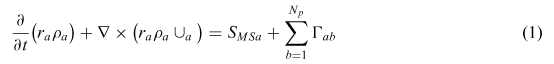Momentum Equations: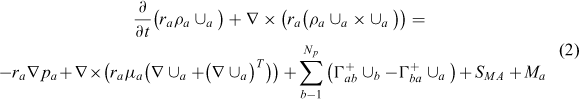where: ra is the volume fraction of each phase (phases indicated by "a", with "a" assuming values from 1 to Np, the total number of phases), pa is the density of phase "a",is the velocity vector of phase "a",is the viscosity of phase "a", SMSa represents mass sources specified by the user;is the mass flow rate per unit volume from phase "b" to phase "a". This term only occurs if interphase mass transfer takes place; SMa represents momentum sources due to external body forces, and Ma represents interfacial forces acting on phase "a" due to the presence of other phases. The term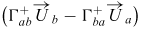represents momentum transfer induced by interphase mass transfer. The volume fractions of the phases sum to unity, that is:There are 4 NP + 1 equations to describe the fluid dynamics for the 5 NP unknowns ra, Ua ||, || Va, Wa, (components of the velocity vectorial field), and Pa (the pressure field of each phase). The additional NP  1 equations needed to close the system of equations were supplied by assuming that all phases shared the same pressure field, P, that is: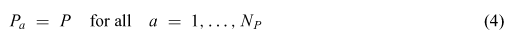A scalar variable Φ in phase "a" has the corresponding transport equation:whereis the Diffusivity of, represents an external source in phase "a", andrepresents sources of Φa due to interphase transfers. Details of the multiphase model may be found in CFX (2004).

To initialize a simulation, a relatively gross mesh was first used to locate the air-water interface. Then, the regions of the upper and lower free surfaces were refined, allowing a detailed location of the interface for the definitive run. The final mesh of each case presented a higher concentration of cells in the upper and lower nappes of the jet, as shown in Figure 4. Details are described by Arantes (2007). Table I shows the main characteristics of the grids and the simulated fluids (air and water). The number of nodes and elements of the grids are mean values for all simulated conditions.

The model allows the adjustment of constants and boundary conditions in order to calibrate the predictions with measured results. Different combinations were tested, but they in general reproduced only

a portion of the observed results. The CFX standard constants and conditions, on the other hand, led to acceptable predictions for the whole set of experiments, being thus used in this study.

The conditions in the inlet were imposed as:

- Pressure: hydrostatic pressure,

- Turbulence intensity: fixed at 5% of the water velocity (standard for CFX),

- Turbulence model: SSG Reynolds Stresses using CFX standard parameters. The model allows the simulation of non-isotropic situations, and the use of the law of the wall.

- Roughness for the law of the wall: 1.0 mm.

The calibration was primarily based on the predictions of the jet lengths.

RESULTS AND DISCUSSION

Fourteen runs were conducted in the chute. Table II provides the experimental conditions. The jet length of run 2 is not available because the jet was longer than the channel. Velocity field measurements using PIV were performed in the nine runs indicated by (*). Results of all fourteen runs were used to check the reproducibility of the data, while the results of the nine runs with velocity data were used to compare with CFD predictions.

COMPARISON OF MEASUREMENTS WITH LITERATURE

Verification of the jet length L

Jet lengths are commonly used to quantify the air uptake by the water flows. The jet lengths of Table II were compared with theoretical predictions of Schwarz andNutt (1963) and Tan (1984), given respectively by equations 6 and 7, and plotted in figure 5. Also shown are the predictions of the empirical correlation of Kökpinar and Gögüs (2002), given by equation 8. The theoretical equations 6 and 7 were obtained with no restrictions to a. Equation 8 was adjusted from experimental data, being thus restricted by the range of a tested during the experiments. A systematic underestimation of equation 8 is observed, which may be due to extrapolations of its limits of application. For example, the ramp slope is limited to 0 < α < 9.45°, but in this study α = 10°.

The good agreement between measured and literature results is taken as an indication that the experimental data for jet length can be used to compare with CFD simulations.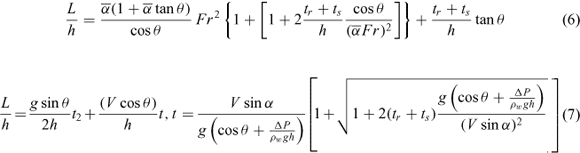In the above equations, α is the ramp slope, θ is the chute slope,is the take off angle (which is the angle followed by the liquid when it leaves the ramp.is equal to α because no inertial effects altered the observed direction of the flow after leaving the ramp), tr is the ramp height, ts is the step height (zero in the present experiments), t is the time, pw is the water density, g is the gravity acceleration ΔP is the relative pressure under the jet (taken positive), h, V, Fr and L are defined in Table I, and Aair/Aw is the ratio between the air supply pipe entrance area, Aa, and the area of the water flow before the aerator, Aw.

Verification of the air entrainment coefficient β as a function of L / h

Pinto et al. (1992) proposed that the ratio between Qair and Qw||, ||Qair/Qw, is proportional do L/h, with a coefficient of proportionality between 0.023 and 0.033. Rutschmann and Hager (1990) found a coefficient of proportionality of 0.030, for the maximum value of β (zero relative pressure in the cavity under the jump). The authors showed that the straight line of β vs. L/ h intercepted the L/ h axis around L / h = 5 for a first set of data, so that the equation β = 0.030(L/ h  5) was proposed. For a second set of data, the simple proportionality β = 0.030L/ h was followed. The authors measured L/ h up to around 45. Chanson (1991) suggested that β and L / h follow linear trends with lower slopes for L / h < 20 and higher slopes for L/h > 20. The L/ h values ranged up to around 25. Kõkpinar and Gõgüs (2002) presented the empirical correlationin which the coefficient of β = β(L/h) depends on the channel slope (θ) and the ratio Aair/Aw. L/h ranged up to 30. Equation 9 shows a nonlinear dependence between β and L/h, and that the coefficient may be lower than 0.0189. The equation is valid for 0 < 9 < 51.3°.

Figure 6 shows β against L / h for the data of these experiments. A constant β seems to occur for L/h between 10 and 15. However, as no measurements were made for L/h < 10, this apparent constancy may be only the scatter of the data. Figure 6 also shows the region containing the lower nappe data of Low (1986) and analyzed by Chanson (1991), obtained for a chute slope θ = 52.33°; and the equations of Rutschmann and Hager (1990), for θ = 51.3° and θ = 34.4°. The dependence of the function β = β(L/h) on geometrical characteristics, such as the slope of the spillway, is clearly visible. Applying equation 9 for the conditions of this study (θ = 14, 5°) led to good predictions of the measured data, with deviations occurring for the lower range of L / h. These deviations may be due to the extrapolations of the application limits of equation 9 or bias in the measurements. As already mentioned, the used ramp slope α = 10° extrapolates the 0 < α < 9.45° limits. Also the experimental range of Froude numbers (Fr), 4.08 < Fr < 15.5, extrapolates the application limits of equation 4, given as 5.56 < Fr < 10.00. However, equation 9 shows that the data followed the general expected behavior for the applied experimental conditions, indicating that the experimental data for air/water flow ratio can be used to compare with CFD simulations.

Verification of the air entrainment coefficient f as a function of Fr

Figure 7 shows the β data of the present study (white circles) and those of Kökpinar and Gögüs (2002) (gray circles) against the Froude number. The data of Kökpinar and Gögüs were obtained for flows over a step height ts of 5 cm, while, for the present data, ts = 0. Moreover, their tr / h values ranged from 0.0 to 0.4, while in the present study they ranged from 0.4 to 1.2. The different experimental conditions generated clouds of points with similar trends, but separated in the graph β vs. Fr, as shown in Figure 7.

To consider the different parameters in a correlation for β, a dimensional analysis leads toThe combined effect of tr and ts is represented here through the ratio ( tr + k • ts)/ h, where k is an adjusted constant. A multiple regression analysis furnished the result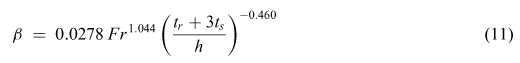Predicted and measured data are presented in Figure 8, showing that the present data and those from literature are complementary and form a single cloud of points. The agreement between present and literature data (Figs. 5, 6 and 8) allowed the use of the corresponding experimental results of air concentration profiles, pressure profiles, velocity profiles and air discharges to compare to the CFD predictions.

COMPARISON OF CFD PREDICTIONS WITH EXPERIMENTS

Jet length

Table III shows six predictions of the jet length. As mentioned, the turbulence conditions were adjusted in the inlet of the numerical domain, but the CFX standard parameters of the turbulence model furnished the best predictions for the set of jet lengths. These conditions were similar for all runs. The simulated lengths were in the range of 0.81 to 1.08 times the measured length for the six runs. These runs were used to obtain numerical air discharges.

Pressure distribution

The pressure distribution along the channel bed was used to measure the jet length, taken as the position of the pressure peak. Figure 9 presents an example measurement and simulation results for run 13, showing good agreement between CFD simulations and the experiment.

Velocity distribution

The velocity distribution in section S3, measured using the PIV technique, was compared with the predicted distribution. Figure 10 shows the comparison for runs 3 and 5, taken as examples. The agreement between measured and predicted profiles is considered acceptable, having a maximum difference often percent. The imposed turbulence intensity in the inlet of the numerical domain, maintained constant for all simulations, may be a cause of the observed deviations. No numerical bias of the related concentration fields were observed (see Fig. 11), so that the obtained velocity distributions were considered adequate for this study.

Concentration profiles

Lima et al. (2008) presented measured values for the air discharge entering into the cavity under the jet, and also values obtained calculating the air discharge from air concentration profiles measured at cross sections along the lower nappe of the jet. The authors applied Equation (12) along sections 4 through 8 (Fig. 2), corresponding to a distance between 3.9% and 132% of the jet lengths.where Qx is the air discharge, B is the channel width, C is the air concentration (void ratio), Vx is the velocity in the x direction and y is the distance normal to the chute face. The upper and lower boundaries of the domain were set at two conditions: 1) C values of 95% and 5%, and 2) C values of 90% and 10%.

Lima et al. (2008) showed that the measured air discharges are substantially lower than the air discharges evaluated from the concentration profiles. Previous results of Wilhelms and Gulliver (2005), when evaluating entrained air from concentration profiles for upper surface aeration, showed that corrections must be made because the measurements involve air among water parcels of the surface distortions, not absorbed by the water. Lima et al. (2008) suggested that a similar effect influenced the measurements along the lower nappe, with the addition of the effect of the spray that emanates from the aerator lip as water. Substantial sprays could account for water measurement throughout the air pocket, affecting the air discharge results.

Numerical predictions of air concentrations are shown in Figure 11. Run 5 is presented because it represents the worst agreement between the measured and predicted jet lengths (predicted length = 81% of measured length). Even for this condition, the agreement between measured and predicted concentration profiles is good. The displacement observed between profiles, mainly for sections S7 and S8, is due to the lower predicted position of the jet when compared with the measured position. Measurements and predictions considered the entire thickness of the jet.

All profiles of Figure 11 were obtained upstream of jet reattachment with the channel bed. Figure 12a shows the predicted concentration field along the jump. Figures 11 and 12 show that the concentration profiles of the upper and lower nappes interact downstream of the position indicated by the black arrow in Figure 12a, where the concentration in the water core ceases to be zero (shown in black). Non-zero air concentrations in the core are related to bubbles originating either at the upper or at the lower surfaces. Figure 12b shows a sketch of an eventual intrusion of air originated in the upper nappe. In this case, concentration measurements can also be affected by this new air source, and air discharges obtained from concentration measurements can eventually be different from those measured in the inlet structure.

The measured and calculated concentration profiles for the impact region could also be compared for one run of the present set of experiments. Figure 13 shows the results obtained for run 12 at section 8, the only case in which the pressure point that quantifies the jet length coincided with a measurement section. Although the general behavior of both profiles is the same, substantial differences are observed between the concentration values. The measured profile is, in general, steeper than the calculated profile, and the concentration differences are higher for distances to the channel bottom between 0 and 5 cm. Also in this case, the differences may result from the turbulence condition imposed in the inlet of the simulation domain. For example, higher levels of homogenization (softer profiles) are obtained for higher turbulent diffusivities, which are dependent on the turbulent conditions.

Air discharges

Predicted air discharges are compared with measurements for the six simulated cases presented in Figure 14. Although the predicted air discharges have the same magnitude as the measured air discharges, a relatively high spread is observed. The predicted air discharges were obtained by integrating air concentration at the cross section of the inlet tube (Fig. 2). The differences between experimental and numerical data are probably associated with the constant parameters of the turbulence model (the CFX standards were used) for the different experimental situations, and the constant turbulence intensity in the inlet of the numerical domain (5%), as previously mentioned. As shown by Ervine et al. (1995), the air uptake by the water depends on the turbulence intensity perpendicular to the flow direction

The results indicate that, for a first evaluation of the magnitude of the air discharge, numerical procedures can be used to help in the decision-making process. However, the numerical code must be calibrated, based on measured characteristics. In the present study, the jet lengths were used to calibrate the model (to adjust parameters and inlet conditions), and the measured concentration, pressure, and velocity profiles were used to check the numerical results. If more detailed information is needed, say for cavitation erosion potential, it is still necessary to conduct experimental studies and to scale up the results based on empirical procedures.

CONCLUSIONS

Experimental studies on air absorption by flows over a bed aerator were described and compared with theoretical and empirical predictions found in the literature and computational results conducted here.

1. Jet lengths were well predicted by the equations of Schwarz andNutt (1963) and Tan (1984). The air entrainment coefficients f, when expressed as a function of

L / h, were well predicted by the equation of Kõkpinar and Gõgüs (2002), unless for the lower range of

L/ h, that is, for ~ 10 <

L/ h <~ 15. In this case, it must be remembered that the conditions of the experiments extrapolated the conditions prescribed by Kõkpinar and Gõgüs (2002). Considering the dependence of the air entrainment coefficient on the Froude number and geometrical characteristics, it was shown that the experimental data here described and those of Kökpinar and Gögüs (2002) can be presented together, following the same trend.

2. Computational predictions of the jet length were taken as control parameter for the numerical simulations (calibrations). The CFX standard parameters were adopted for the turbulence model. The turbulence intensity at the inlet was fixed at 5% of the mean flow velocity, and the roughness used in the law of the wall was fixed at 1.0 mm, which generated predicted jet lengths between 0.8 and 1.2 times the measured length.

3. It was observed that the pressure distribution along the channel bed, the velocity profiles at the end of the ramp, and the concentration profiles along the jet could be represented by the calibrated computational code. Considering the air concentration at the impact point, the measured and predicted profiles presented the same general form, although concentration differences were observed.

4. The computational predictions of the air discharges followed the magnitude of the measured data. However, a relatively large scatter was observed. For a first evaluation of the magnitude of the air discharge, computational procedures can be used to help in making decisions. However, the numerical code must be calibrated, based on previous measured characteristics. In the present study, the jet lengths were used to calibrate the model (to adjust parameters and inlet conditions), and the measured concentration, pressure, and velocity profiles were used to check the numerical results.

ACKNOWLEDGMENTS

The authors thank Profs. M.A. Kökpinar and M. Gögüs, who furnished the β data used in Figures 7 and 8; Coordenação de Aperfeiçoamento de Pessoal de Nível Superior (CAPES - pr. 2201/06-2) and Fundação de Amparo à Pesquisa do Estado de São Paulo (FAPESP), for supporting this research. The authors do not have any propriety and financial interest in any product or company cited in this manuscript.

Manuscript received on September 14, 2008; accepted for publication on September 30, 2009

• ARANTES EJ. 2007. Caracterização do Escoamento sobre Vertedores em Degraus via CFD. Tese de Doutorado, Escola de Engenharia, Universidade de São Paulo, São Carlos, Brasil, 204 p.
• BORSARI RD. 1986. A cavitação do Escoamento sobre Vertedores em Degraus via CFD. Tese de Doutorado, Escola de Engenharia, Universidade de São Paulo, São Carlos, Brasil, 246 p.
• CARVALHO PD. 1997. Aeração de Escoamentos de Alta Velocidade em Canais de Forte Declividade. Tese de Doutorado, Escola de Engenharia, Universidade de São Paulo, São Carlos, Brasil, 387 p.
• CFX. 2004. CFX 5 Solver Theory. Ansys Canada Ltda, Waterloo, ontario, Canada, 250 p.
• CHANSON H. 1991. Aeration of a Free Jet Above a Spillway. J Hydraul Res 29(5): 655-667.
• ERVINE DA, FALVEY HT AND KAHN AR. 1995. Turbulent Flow Structure and Air Uptake at Aerators. Hydro-power and Dams, September, 89-96.
• FUENTES R. 1992. Modelación Física en Hidráulica (Physical Modeling in Hydraulics), Actas del curso pré-congreso del XV Congreso Latinoamericano de Hidraulica, IAHR, Universidad de Cartagena, Colômbia.
• KÖKPINAR MA AND GÖGÜS M. 2002. High-speed Jet Flows Over Spillway Aerators. Can J Civ Eng 29: 885-898.
• LIMA ACM. 2004. Caracterização da estrutura turbulenta em escoamentos aerados em canal de forte declividade com auxílio de técnicas de velocimetria a laser. Vols. 1 e 2. Tese de Doutorado, Escola de Engenharia, Universidade de São Paulo, São Carlos, Brasil.
• LIMA ACM, SCHULZ HE AND GULLIVER JS. 2008. Air uptake in the lower nappe of a spillway aerator. J Hydraulic Res 46(6): 839-843. doi:10.3826/jhr.2008.3296.
• LOW HS. 1986. Model Studies of Clyde Dam Spillway Aerators. Master Report, Ref 86-6, University of Canterbury, New zealand.
• PAN S, SHAO Y, SHI Q AND DONG X. 1980. The Self-aeration Capacity of the Water Jet Over the Aeration Ramp. J Hydr Engrg (Shuili Xuebao - in Chinese with abstract in English), Beijing, PRC, 5, Oct, 13-22.
• PINTO NL DE S, NEIDERT SH AND OTA JJ. 1982. Aeration at High Velocity Flows. Water Power and Dam Constr 34-38: 42-44.
• RUTSCHMANN P AND HAGER WH. 1990. Air Entrainment by Spillway Aerators. J Hydr Engrg 116(6): 765-782.
• SCHWARZ I AND NUTT LP. 1963. Projected Nappes subjected to Transverse Pressure. J Hydr Div ASCE 89(7): 97-104.
• TAN P. 1984. Model Studies of Aerators and Spillways. Research Report 84-6, Dept Civil Engg,Univ of Canterbury, Christchurch, New Zealand.
• TOOMBES L AND CHANSON H. 2005. Air Entrainment and Velocity Redistribution in a Bottom Outlet Jet Flow. XXXIIAHR Congress, September 11 to 16, Seoul, Korea, 2716-2726.
• VOLKART P. 1980. The Mechanism of Air Bubble Entrainment in Self-Aerated Flow. Int Jf Multiphase Flow 6: 411-423.
• WILHELMS SC AND GULLIVER JS. 2005. Bubbles and Waves Description of Self-aerated Spillway Flow. J Hydraul Res 43(5): 522-531.
• WOOD IR. 1985. Air Water Flows, Keynote address, Proceedings of the 21th IAHR Congress, Melbourne, Australia, 6: 18-29.
• Correspondence to:
Prof. Harry Edmar Schulz
E-mails:

# Publication Dates

• Publication in this collection
11 June 2010
• Date of issue
June 2010

• Accepted
30 Sept 2009